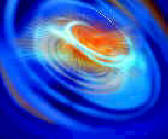#Wordtrade.com Physics

### Review Essays of Academic, Professional & Technical Books in the Humanities & Sciences

Optimization Algorithms in Physics by Alexander K. Hartman and Heikp Rieger (John Wiley & Sons) The past few years have witnessed a substantial growth in the number of applications for optimization algorithms in solving problems in the field of physics. Examples include determining the structure of molecules, estimating the parameters of interacting galaxies, the ground states of electronic quantum systems, the behavior of disordered magnetic materials, and phase transitions in combinatorial optimization problems. This book serves as an introduction to the field, while also presenting a complete overview of modern algorithms. The authors begin with the relevant foundations from computer science, graph theory and statistical physics, before moving on to thoroughly explain algorithms - backed by illustrative examples. They include pertinent mathematical transformations, which in turn are used to make the physical problems tractable with methods from combinatorial optimization. Throughout, a number of interesting results are shown for all physical examples. The final chapter provides numerous practical hints on software development, testing programs, and evaluating the results of computer experiments.

Optimization Algorithms in Physics is an interdisciplinary book: it tries to teach physicists the basic knowledge of combinatorial and stochastic optimization and describes to the computer scientists physical problems and theoretical models in which their optimization algorithms are needed. It is a unique book since it describes theoretical models and practical situation in physics in which optimization problems occur, and it explains from a physicists point of view the sophisticated and highly efficient algorithmic techniques that otherwise can only be found specialized computer science textbooks or even just in research journals. Traditionally, there has always been a strong scientific interaction between physicists and mathematicians in developing physics theories. However, even though numerical computations are now commonplace in physics, no comparable interaction between physicists and computer scientists has been developed. Over the last three decades the design and the analysis of algorithms for decision and optimization problems have evolved rapidly. Most of the active transfer of the results was to economics and engineering and many algorithmic developments were motivated by applications in these areas.

The few interactions between physicists and computer scientists were often successful and provided new insights in both fields. For example, in one direction, the algorith­mic community has profited from the introduction of general purpose optimization tools like the simulated annealing technique that originated in the physics community. In the opposite direction, algorithms in linear, nonlinear, and discrete optimization sometimes have the potential to be useful tools in physics, in particular in the study of strongly disordered, amorphous and glassy materials. These systems have in common a highly non‑trivial minimal energy configuration, whose characteristic features dom­inate the physics at low temperatures. For a theoretical understanding the knowledge of the so called "ground states" of model Hamiltonians, or optimal solutions of appro­priate cost functions, is mandatory. To this end an efficient algorithm, applicable to reasonably sized instances, is a necessary condition.

The list of interesting physical problems in this context is long, it ranges from disor­dered magnets, structural glasses and superconductors through polymers, membranes, and proteins to neural networks. The predominant method used by physicists to study these questions numerically are Monte Carlo simulations and/or simulated annealing. These methods are doomed to fail in the most interesting situations. But, as pointed out above, many useful results in optimization algorithms research never reach the physics community, and interesting computational problems in physics do not come to the attention of algorithm designers. We therefore think that there is a definite need to intensify the interaction between the computer science and physics communities. We hope that this book will help to extend the bridge between these two groups. Since one end is on the physics side, we will try to guide a number of physicists to a journey to the other side such that they can profit from the enormous wealth in algorithmic techniques they will find there and that could help them in solving their computational problems.